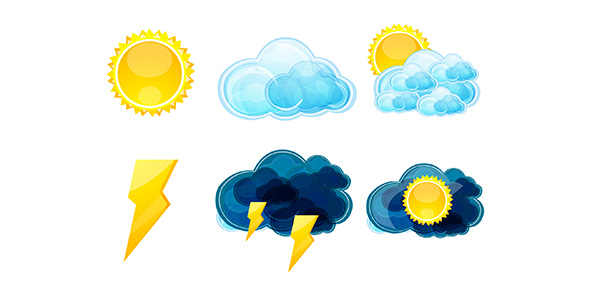Systems And Models: The Water Cycle Trivia Questions! Quiz

21 QuestionsSettingsWhen it comes to describing a system in science, we usually use model. or charts. It is, therefore, essential for people to know the basics when it comes to explaining or drawing a model. Can explain the water cycle using a model? Take up this test and see just how much you know about the processes involves and how to explain them.

• 1.
A model is a representation of an object, process, or phenomenon?
• A.

True

• B.

False

• 2.
• A.

Something you only see on a clock

• B.

Something that helps you see what happens to data over time

• C.

A variable in all systems

• D.

Something that always goes on the Y axis

• 3.
A ______ is a kind of model and there are two types they are ______ and  ______
• 4.
A _____ system does not share matter but share energy in and out of a system
• 5.
A _____ system shares energy and matter
• 6.
4% of hydrosphere is freshwater
• A.

True

• B.

False

• 7.
Select all the different ways water enters and leaves the water cycle.
• A.

Evaporation

• B.

Condensation

• C.

Precittation

• D.

Boiling water

• E.

Run off

• F.

Groundwater

• G.

Out of a hose

• H.

Storage

• I.

Respiration/breathing

• J.

Throwing water into the air

• K.

Transpirtation

• L.

Evapotranspiration

• 8.
Describe the carbon cycle.
• 9.
_____ thought that if you think it it is, and ______ thought that you have to test before you claim things.
• 10.
Earth is a _____ system
• 11.
Check all of the graphing steps
• A.

Identify the variables

• B.

Determine scale

• C.

Determine the range

• D.

Number and label each axis

• E.

Plot

• F.

Draw

• G.

Give graph title

• 12.
The ___ variable is what you changed and goes on the x axis
• 13.
The _____ variable changes with the other variable and goes on the y axis
• 14.
Describe the Law of Thermodynamics
• 15.
The carbon cycle can enter the atmosphere by, breathing out, burning of trees and bush, volcanoes, diffusion out of oceans, decomposers
• A.

True

• B.

False

• 16.
• A.

If- dependent then- independent

• B.

If- independent then- dependent

• C.

Idk

• D.

Neither

• 17.
_____ is a logical interpretation of an event that is based on observations and prior knowledge
Related Topics